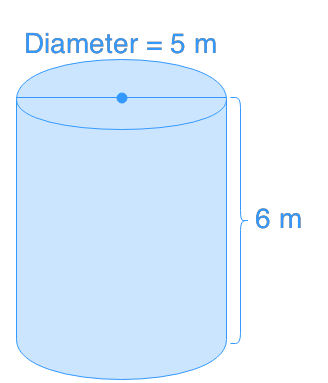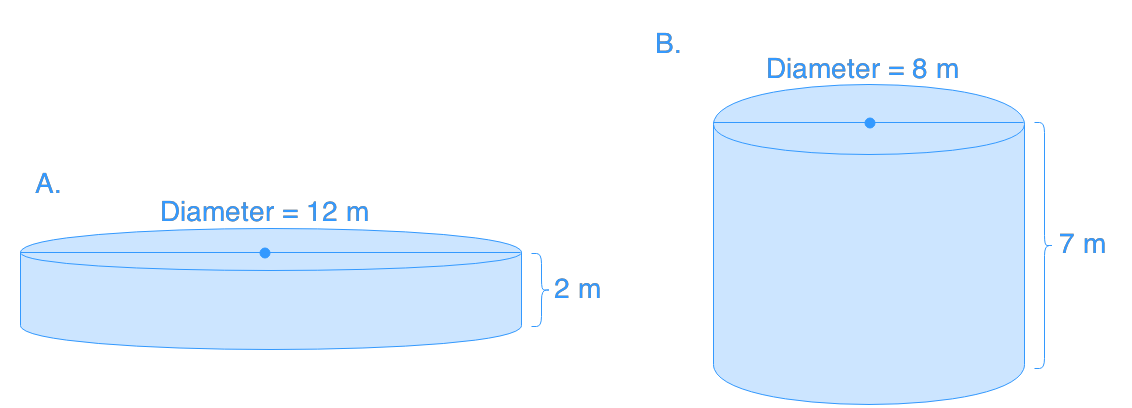# Surface area of cylinders

### Surface area of cylinders

As long as we have the diameter or radius and the height of the cylinder, we are able to find its surface area in no time. The surface area of a cylinder is basically adding the surface are of a rectangle and two circles together. The most difficult part is to find the circumference of the circles, which is also one of the sides of the rectangle.

#### Lessons

• Introduction
a)
• What is Area?
• What is Surface Area?
• How to find the Area of a Cylinder?

• 1.
Determine the surface area of the cylinder.
a)
height: 7 cm, radius: 3 cm

b)
height: 12 cm, radius: 3 cm

• 2.
Find the surface area of the tube below.• 3.
Find the surface area of the tube below.a)
Which cylinder has a larger surface area?

b)
Change the height of cylinder A so that cylinders A and B will have the same surface area.